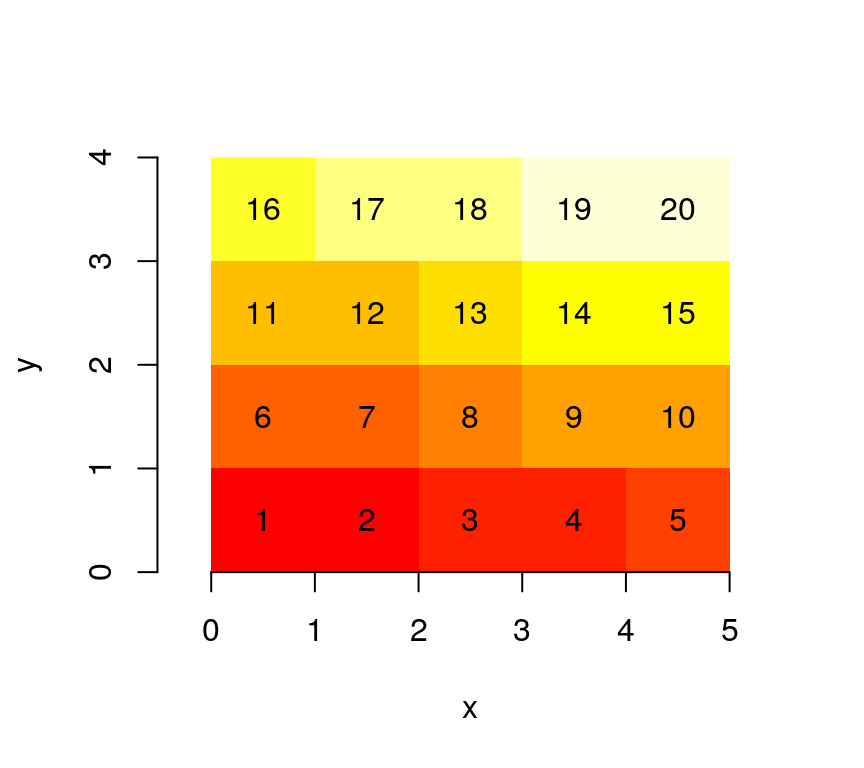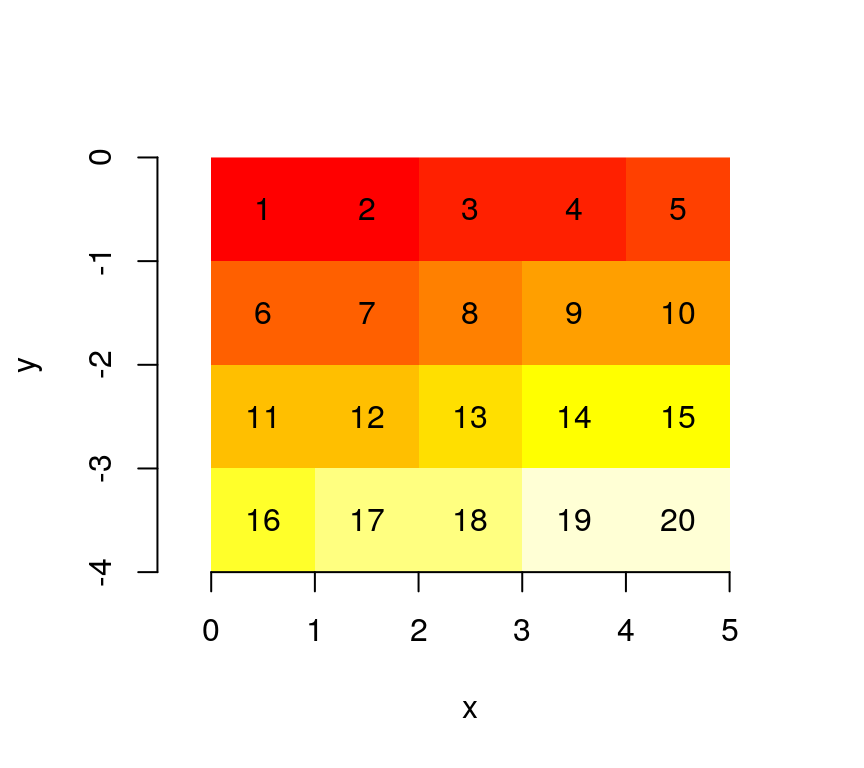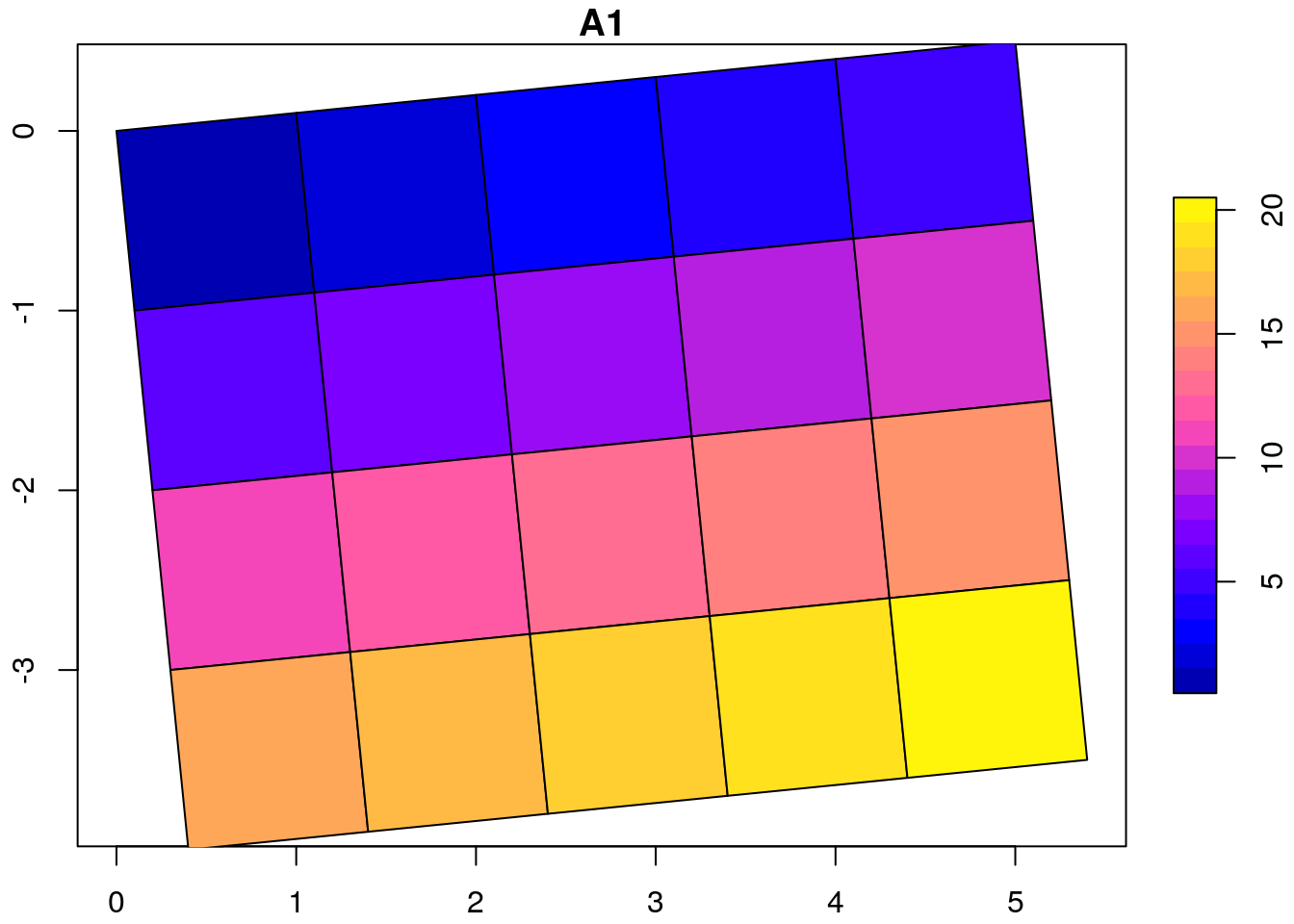This vignette explains the data model of stars objects, with illustrations.

## Grid types

### Regular grids

With a very simple file created from a $$4 \times 5$$ matrix

suppressPackageStartupMessages(library(stars))
m = matrix(1:20, nrow = 5, ncol = 4)
dim(m) = c(x = 5, y = 4) # named dim
(s = st_as_stars(m))
## stars object with 2 dimensions and 1 attribute
## attribute(s):
##       A1
##  Min.   : 1.00
##  1st Qu.: 5.75
##  Median :10.50
##  Mean   :10.50
##  3rd Qu.:15.25
##  Max.   :20.00
## dimension(s):
##   from to offset delta refsys point values
## x    1  5      0     1     NA    NA   NULL
## y    1  4      0     1     NA    NA   NULL

we see that

• the rows (5) are mapped to the first dimension, the x-coordinate
• the columns (4) are mapped to the second dimension, the y-coordinate
• the from and to fields of the dimensions are redundant, as they also are present in the array dimension:
dim(s[])
## x y
## 5 4
• offset and delta specify how increasing row/column index maps to x and y coordinate values

When we plot this object, using the image method for stars objects, we see

image(s, text_values = TRUE, axes = TRUE)Where it becomes clear that $$(0,0)$$ is the origin of the grid (grid corner), and $$1$$ the coordinate value increase from one index (row, col) to the next. It means that consecutive matrix columns represent grid lines, going from south to north. Grids defined this way are regular: grid cell size is constant everywhere.

Most grid data comes with y coordinates (grid rows) going from North to South (top to bottom); this is established using a negative delta. We see that the grid origing $$(0,0)$$ did not change:

attr(s, "dimensions")$y$delta = -1
attr(s, "dimensions")$x$geotransform = -1
attr(s, "dimensions")$y$geotransform = -1
image(s, text_values = TRUE, axes = TRUE)An example is the GeoTIFF carried in the package, which has a negative delta for the y-coordinate:

tif = system.file("tif/L7_ETMs.tif", package = "stars")
##   from  to  offset delta                       refsys point values
## y    1 352 9120761 -28.5 +proj=utm +zone=25 +south... FALSE   NULL

### Regular grids: rotated

x and y coordinate grids carry a geotransform field, used to compute $$x$$ and $$y$$ coordinates from grid index $$i$$ and $$j$$:

$x = gt_1 + (i-1) gt_2 + (j-1) gt_3$

$y = gt_4 + (i-1) gt_5 + (j-1) gt_6$ We can rotate grids by setting $$gt_3$$ and $$gt_5$$ to a constant, non-zero value:

attr(s, "dimensions")$x$geotransform[c(3,5)] = 0.1
attr(s, "dimensions")$y$geotransform[c(3,5)] = 0.1
# FIXME: use image()
plot(st_as_sf(s, as_points = FALSE), axes = TRUE, nbreaks = 20)The rotation angle, in degrees, is

atan2(0.1, 1) * 180 / pi
##  5.710593

### Regular grids: sheared, sheared and rotated

Sheared grids are obtained when the two rotation coefficients, $$gt_3$$ and $$gt_5$$, are unequal:

attr(s, "dimensions")$x$geotransform[c(3,5)] = c(0.1, 0.2)
attr(s, "dimensions")$y$geotransform[c(3,5)] = c(0.1, 0.2)
plot(st_as_sf(s, as_points = FALSE), axes = TRUE, nbreaks = 20)